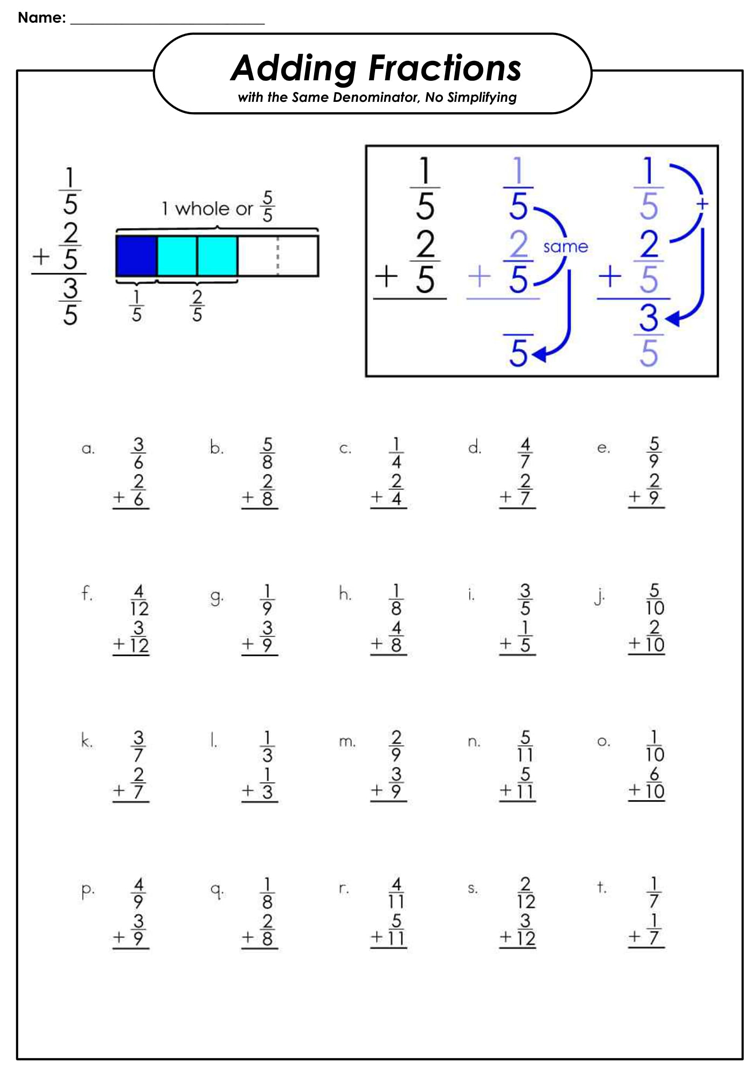# Fraction Worksheets for Practice (Grade 3-6)

To assist your child gain and learn more skills about fractions, it is important to use fraction worksheets. They normally cover crucial skills such as multiplying, simplifying fractions and subtracting fractions. They are important math concepts that your child needs to learn in grade five to create a solid foundation for complex problems at higher grades.

With the correct method of teaching, fractions can be fun and easy to learn for children. The first thing is to make sure the students understand what fractions are and how they work. This is well achieved by use of worksheets with fraction circles or manipulative. Ensure to first work with like denominators then shift to unlike denominators when the students have learned the basic concept of fractions.

There are several sets of fraction worksheets that teachers can use to educate students about fractions. Some of them include the following:

## Manipulative Fraction Tools

They consist of Fraction strips which can either be colored or Black and White. These printable fraction strips can be used to teach the concept of fractions as a hands-on way. Comparing fractions and equivalent fractions can also be taught which include halves, thirds, and so on.

## Fraction worksheets of Diagrams and shapes

They consist of diagrams printed on a large sheet, divided using lines and shaded on particular portions. Make the student determine what fraction has been shaded, which is a basic learning method. The particular fractions could be indicated with a missing numerator or denominator for the students to fill out.

## Use of Basic Fraction Games

Children normally like this fraction chain reaction game. It is a memory catching game where a student is required to match the fraction number cards with the fraction picture card.

There are many kinds of Printable Fraction worksheets in which students need to be exposed to answering fraction problems which require thinking. In this case, they have to find the different ways to divide shapes and determine the shapes divided into fractions.

## Worksheet of Comparing Fractions Sample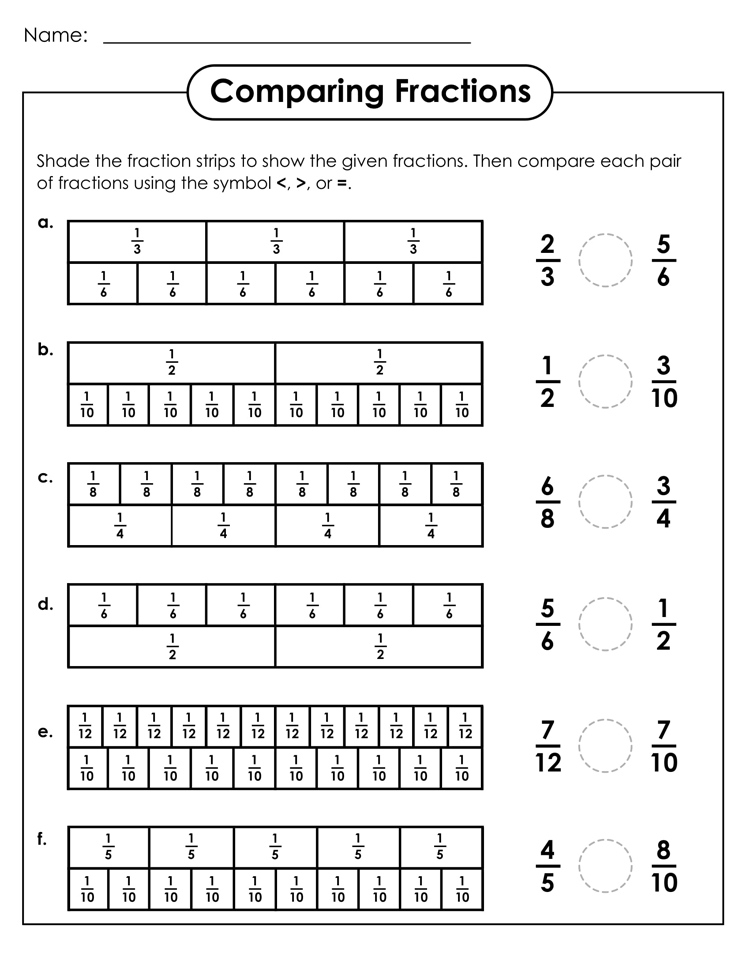## Sample of Fraction Shapes Worksheet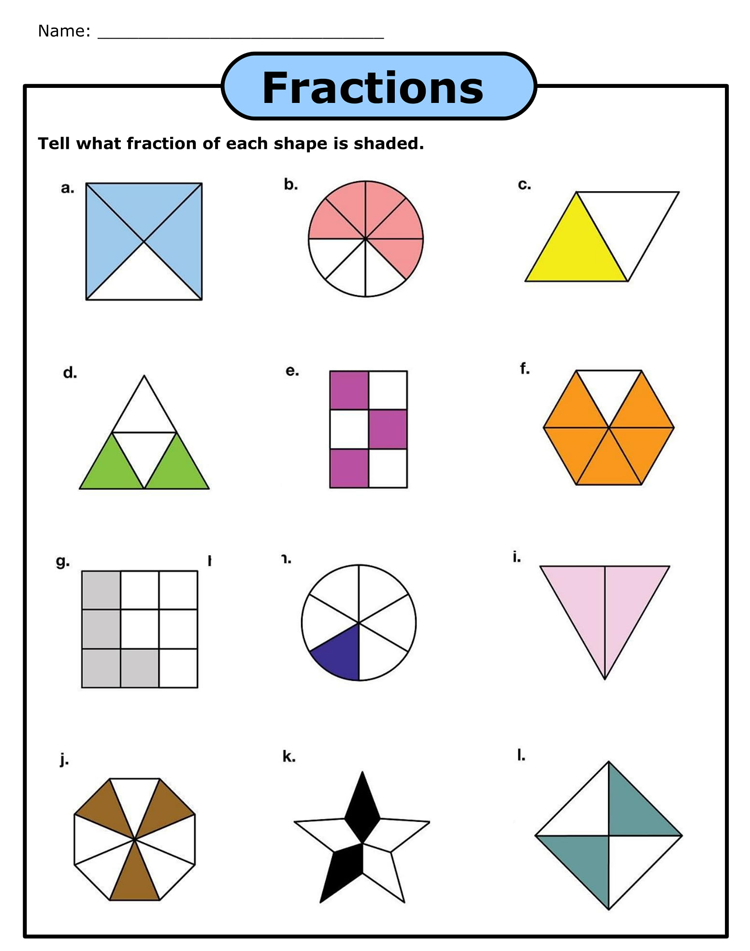## Equal Fractions Match Game Worksheet Example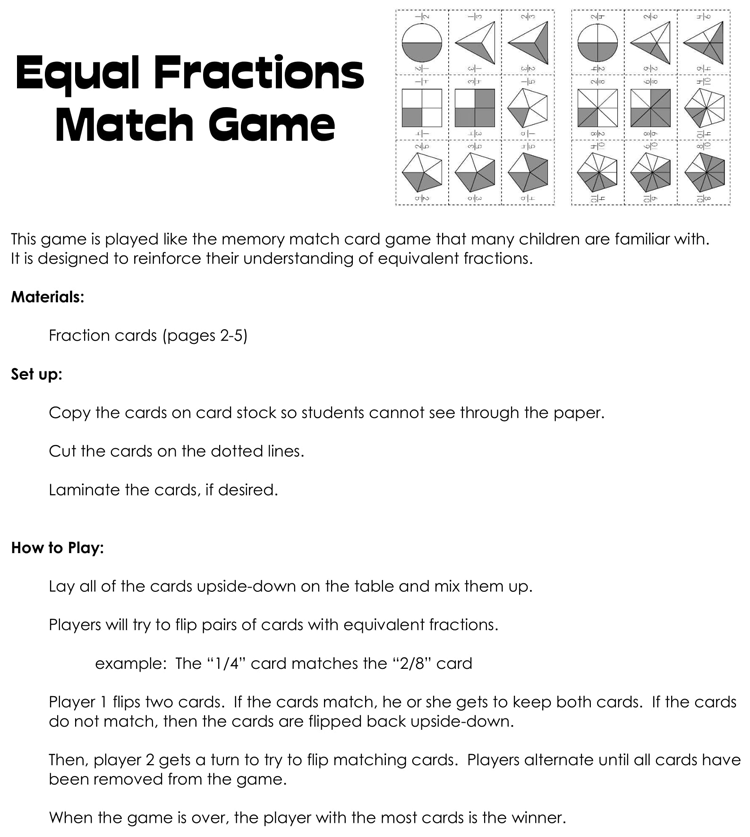## Sample of Subtracting Fractions Worksheet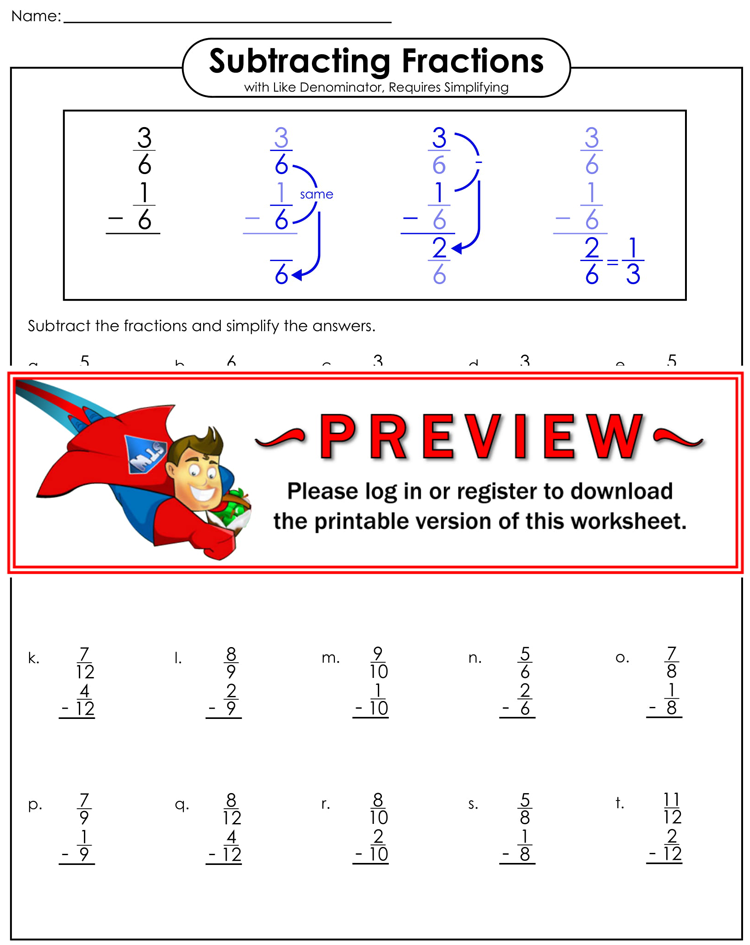## Sample of Adding Fractions Worksheet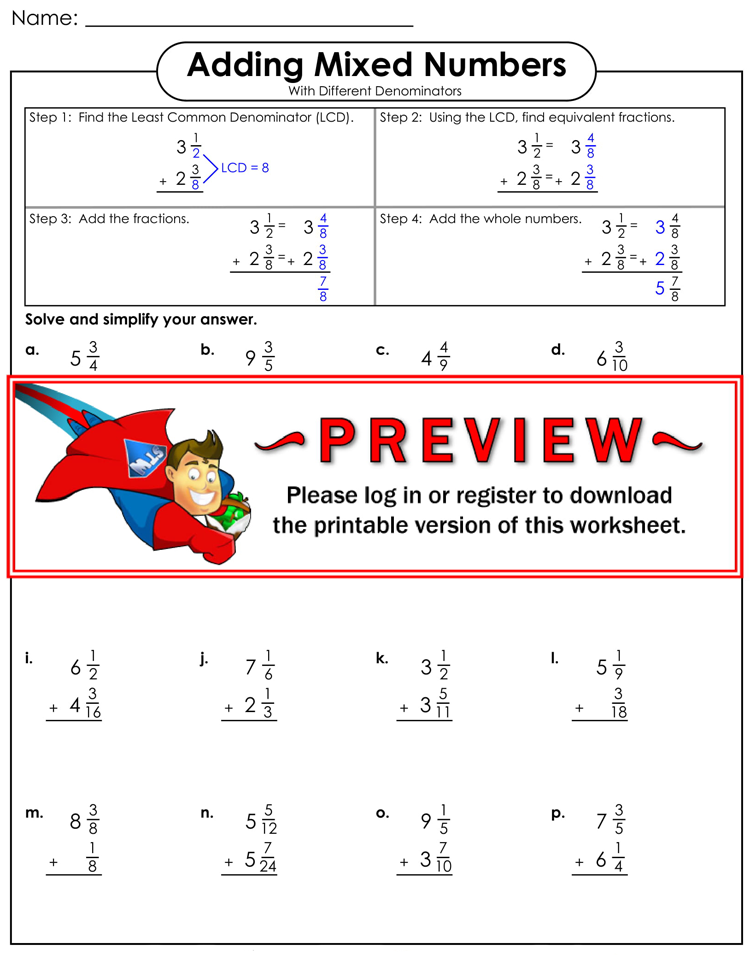## Percentages in Fractions Worksheet Format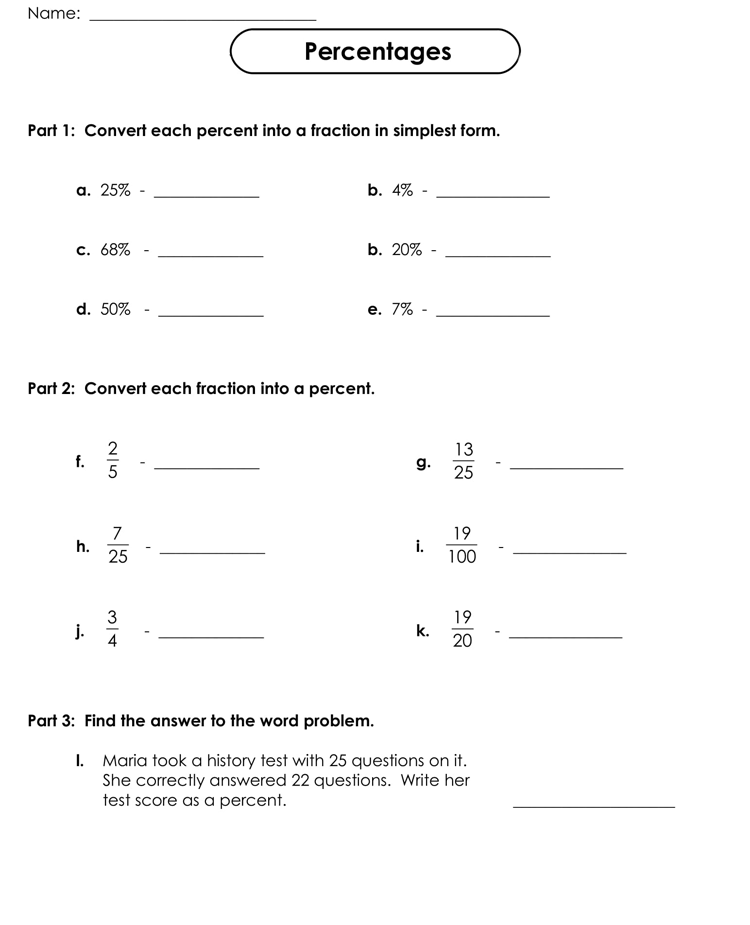## Free Download Fractions of Groups Worksheet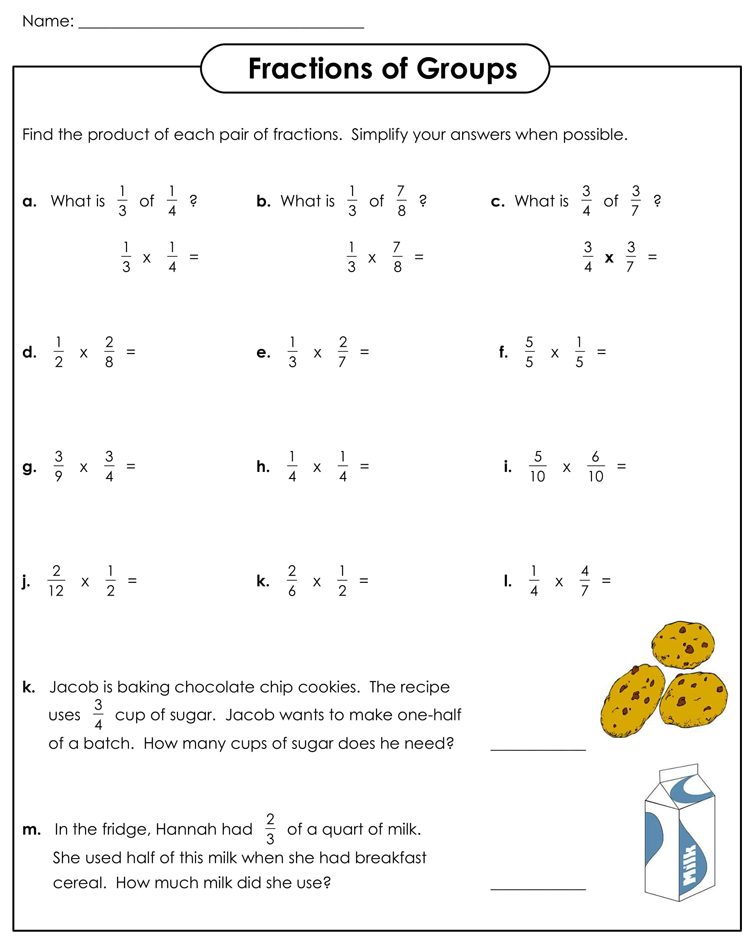## Editable Adding Fractions Worksheet Sample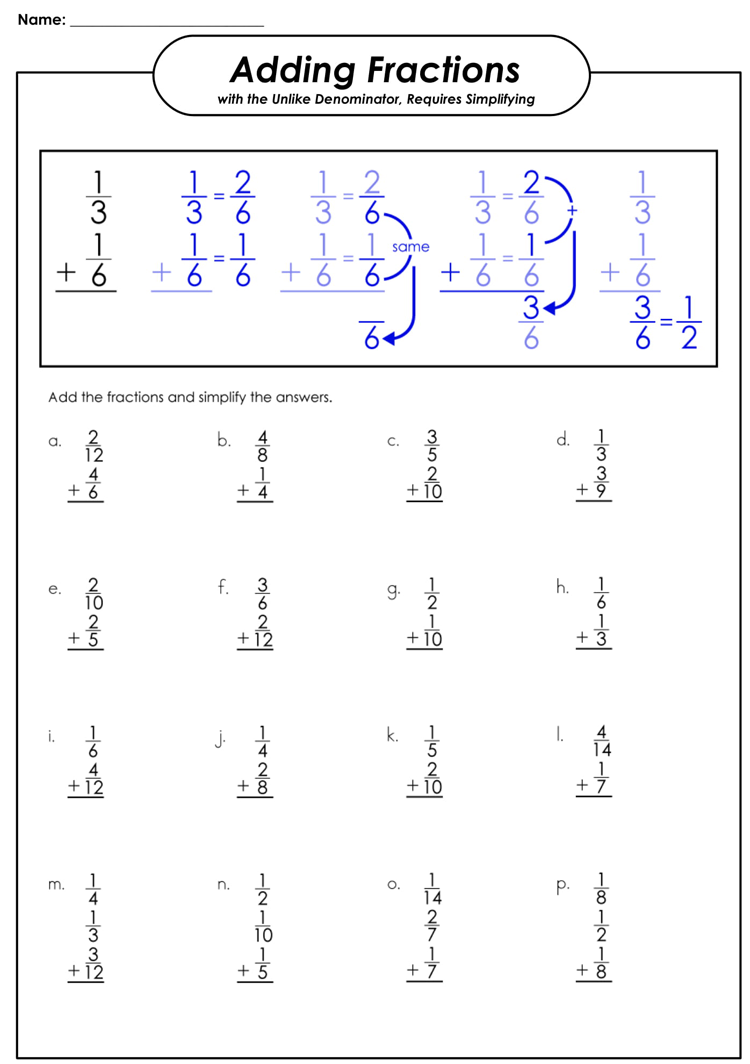## Adding Fractions Worksheet Format